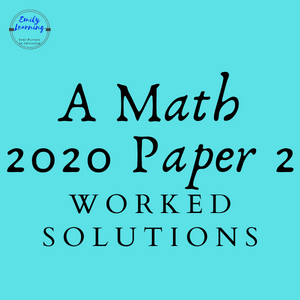# Suggested Solutions: 2020 GCE O Level A Math Paper 2Here, you will find the suggested answer for 2020 GCE O Level A Math exam Paper 2. Click on the link below to go to the question directly.

Question 2

Question 3

Question 4

Question 6

Question 7

Question 8

Question 9

Question 10

## Question 1 on Trigonometry (R- formula)

A very typical R- formula question. Students are first asked to apply R- formula to a trigonometric expression. Next they are asked for the minimum and maximum value, and find the angle at which it occurs.

## Question 2 on Simultaneous equations and Quadratic functions

This question involves solving non-linear simultaneous equations in part (a), followed by testing students on applying discriminants to quadratic functions, given certain scenario.

## Question 3 on Binomial Theorem

In this question, students are expected to make use of the expression for the (r+1)th term of the binomial expansion to solve the question.

## Question 4 on Proofs in Plane Geometry (not tested in 2021 as the common last topic is taken out)

Proofs in plane geometry is taken out from 2021 O Level A math exam. However, I have included the worked solutions.

## Question 5 on quadratic inequalities and sketching of graphs

This is a question on solving quadratic inequalities, and sketching of graphs y = axn.

## Question 6 on linear law

This is a question on linear law. Students are expected to draw a straight line graph for this question.

## Question 7 on Differentiation and Integration

This questions tests students on the concept of integration as a reverse of differentiation. Part (a) involve differentiation, and the rest of the question involves using the results from the differentiation, to solve an integration question.

## Question 8 on Logarithm

A question on logarithm, and a bit on indices.

## Question 9 on Coordinate Geometry

This is a question on coordinate geometry. Students are given a parallelogram, and the coordinates of some points. Concepts tested include finding point of intersection, finding length between two points, finding gradient as well as equation of straight line.

## Question 10 on Integration

This is a question that involves integration of f”(x) to obtain f'(x), and the integrating f'(x) to obtain f(x).

## Question 11 on Rate of Change (Differentiation)

This is a question on using differentiation to find rate of change. Students are also tested on the use of chain rule and integration.

## Learn On-demand – A- Math Courses

If you want to learn a particular topic in detail, check out our on-demand O Level A Math courses here.

Suggested Answers for other A Math Papers: